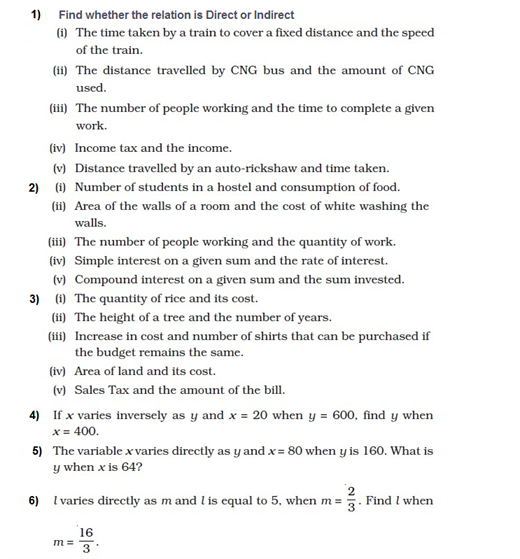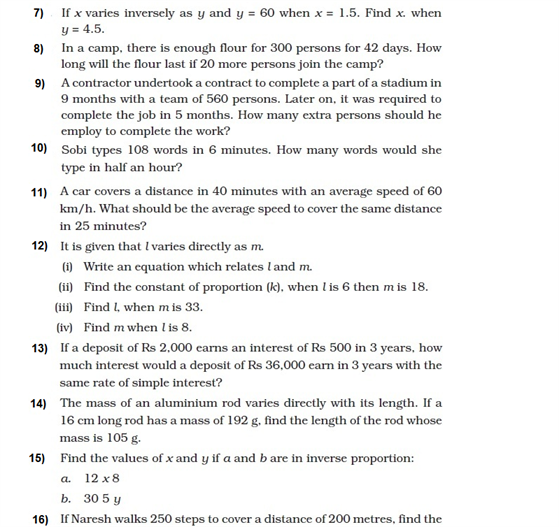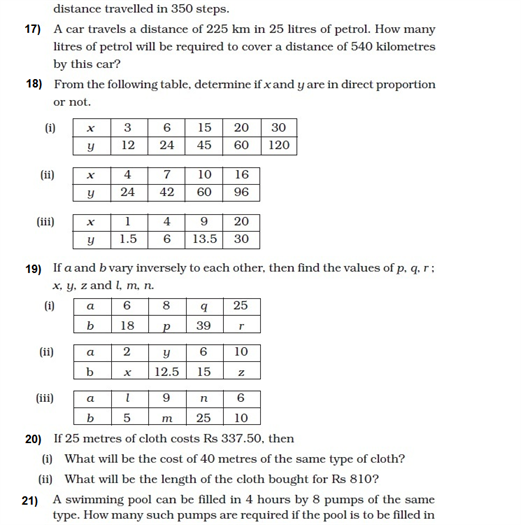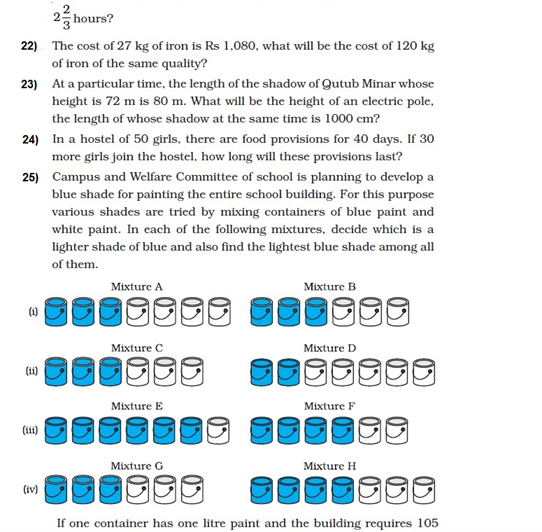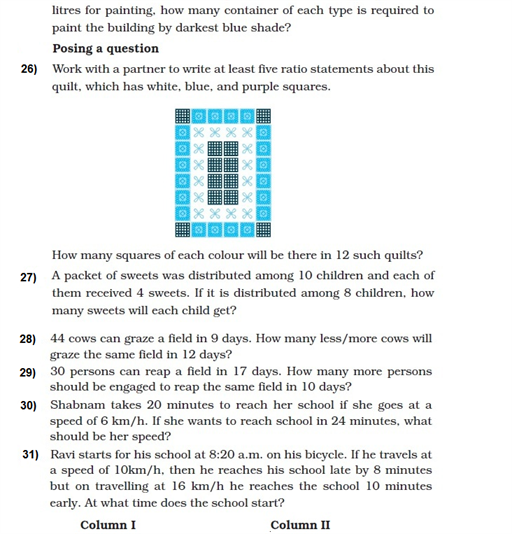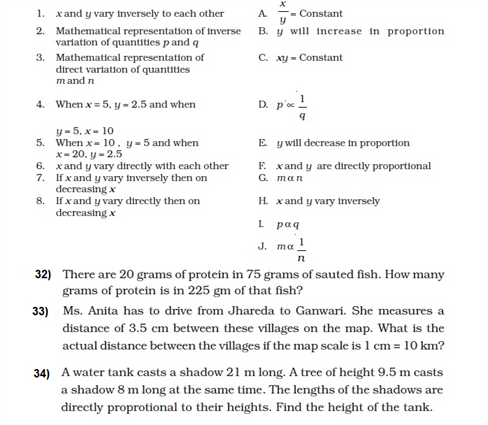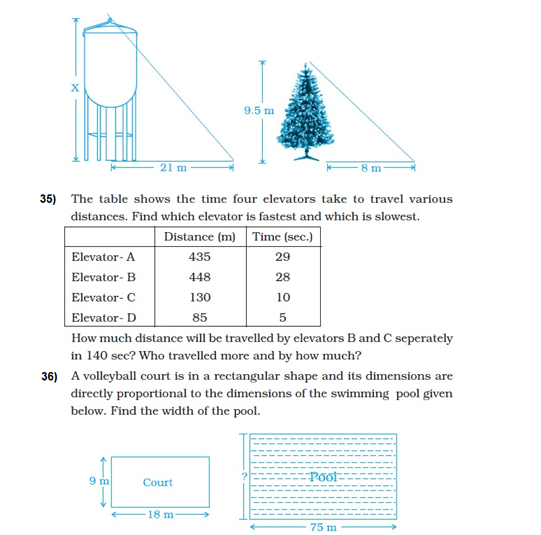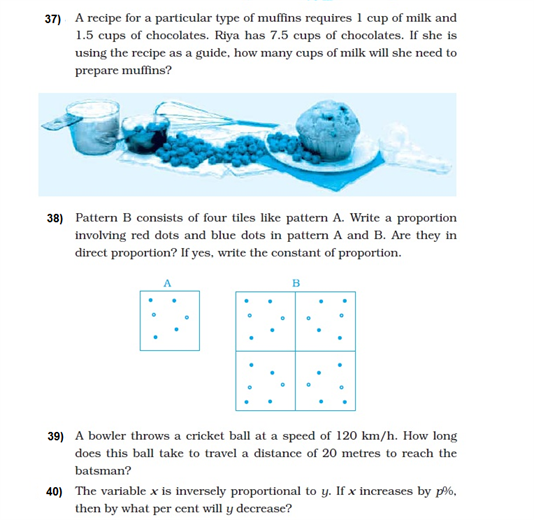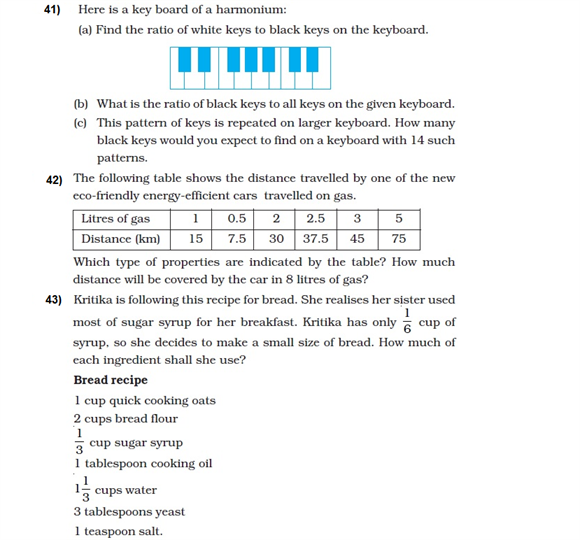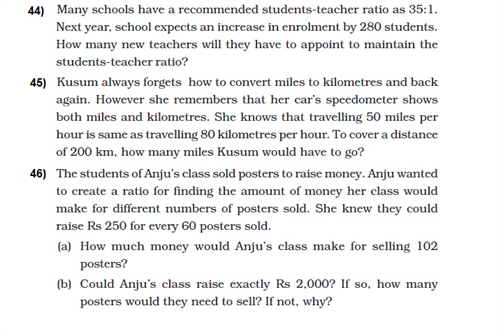# Important Questions Class 8 Maths Chapter 13 Direct and Inverse Proportions

Some important class 8 maths questions for chapter 13 direct and inverse proportions are given here to help the students to get acquainted with different variations of questions and thus, score well in the class 9 CBSE exam. These direct and inverse proportion questions include various long, short and HOTS questions which will also help students to develop their problem-solving skills.

Also Check:

## Direct and Inverse Proportions Questions For Class 8 (Chapter 13)

A few important direct and inverse questions with solutions for class 8 are given below. There are several additional practice questions given below that students can practice once they are completely acquainted with the concepts.

### Important Direct and Inverse Proportion Questions (With Solutions)

1. If the increase in time brings about a corresponding decrease in the value of a product. Identify the proportionality.

Solution:

Here, the increase in time reduces the value of a product. Thus,

Time ∝ 1/Product Value

So, the time and the value of the product are inversely proportional.

2. Identify the variation: “For the increase in speed, the time to cover a fixed distance reduces”.

Solution:

In this case, an increase in speed results in a decrease in time. So,

Speed ∝ 1/Time

So, this relation is a case of indirect variation.

3. If the cost of 20 pens is Rs. 180, calculate the cost of 15 pens?

Solution:

Given that 20 pens cost Rs. 180.

Now, let the cost of 15 pens be Rs. x

In this situation, the cost of pens varies directly with the total number of pens i.e. they are directly proportional.

So,

20/180 = 15/x

Or, x = Rs. 135.

4. A car travels 14km in 25 minutes. Find out how far the car can travel in 5 hours if the speed remains the same?

Solution:

It is given that the car travels 14km in 25 minutes.

Now, assume that the distance the car can travel in 5 hours be x.

Since 1 hour = 60 minutes, 5 hours = 300 minutes.

Thus, the two given statements are

14km —————–> 25 minutes

And, x km —————–> 300 minutes

We know that the distance travelled by car and the time taken by the car is directly proportional to each other.

So,

14/25 = x/300

=> x = 168 km.

5. If 15 workers can finish a task in 42 hours, calculate the number of workers required to complete the same task in 30 hours?

Solution:

In this situation, the number of workers varies directly with the time required to finish a task.

Thus, they are inversely proportional.

Now, assume that the number of workers required to complete the task in 30 hours be “x”.

Here, the number of workers ∝ 1/hours

Or,

Number of workers = C/hours (here “C” is the constant of proportionality)

Now, consider the first case: “15 workers can finish a task in 42 hours”

Here, 15 = C/42

=> C = 15 × 42 = 630.

Now, consider the second case: “x workers can finish a task in 30 hours”

Here, x = C/30

=> x = 630/30

Or, x = 21

So, the number of 21 workers are required to complete the task in 30 hours.

### Extra Questions From Class 8 Direct and Inverse Proportion (Self Assesment)